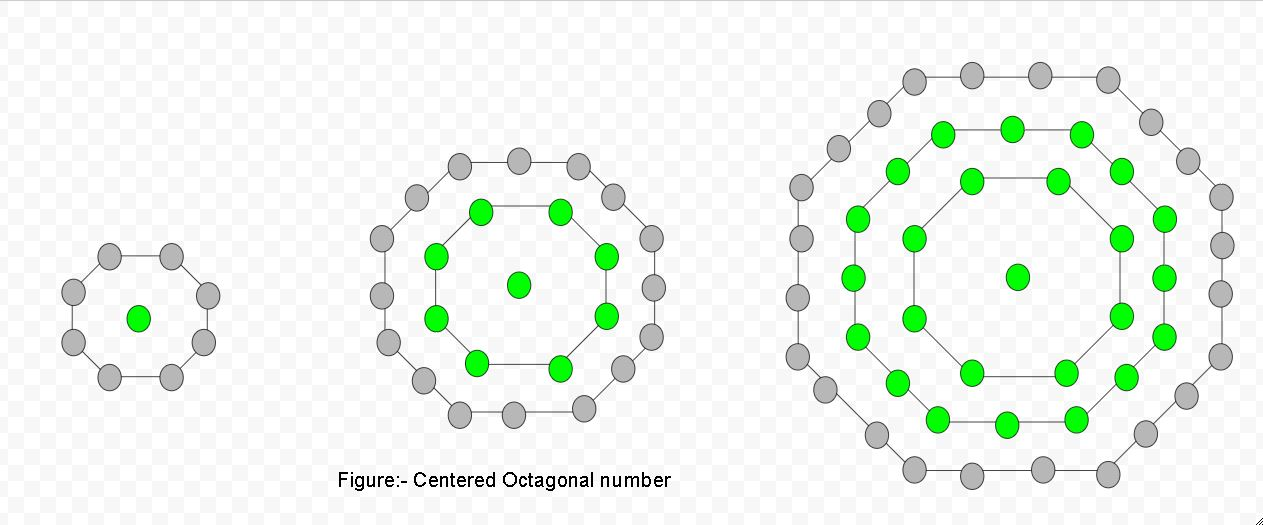# Centered Octagonal Number

Given a number n, find the nth centered octagonal number.

A centered octagonal number represents an octagon with a dot in the centre and others dots surrounding the centre dot in the successive octagonal layer.Examples :

```Input :  2
Output : 9

Input : 5
Output : 81
```

## Recommended: Please try your approach on {IDE} first, before moving on to the solution.

Centered Octagonal n-th Number is given by :

``````

Basic Implementation of the above approach:

## C++

 `// Program to find nth ` `// centered octagonal  ` `// number ` `#include ` `using` `namespace` `std; ` ` `  `// Centered octagonal number function ` `int` `cen_octagonalnum(``long` `int` `n) ` `{ ` `    ``// Formula to calculate nth ` `    ``// centered octagonal number & ` `    ``// return it into main function. ` `    ``return` `(4 * n * n - 4 * n + 1); ` `} ` ` `  `// Driver Code ` `int` `main() ` `{ ` `    ``long` `int` `n = 6; ` `    ``cout << n << ``"th  centered"`  `                 ``<< ``" octagonal number : "``; ` `    ``cout << cen_octagonalnum(n); ` `    ``cout << endl; ` `    ``n = 11; ` `    ``cout << n << ``"th  centered"` `                  ``<< ``" octagonal number : "``; ` `    ``cout << cen_octagonalnum(n); ` ` `  `    ``return` `0; ` `} `

## Java

 `// Java Program to find nth ` `// centered octagonal number ` `import` `java.io.*; ` `  `  `class` `GFG  ` `{ ` `      `  `    ``// Function to find centered ` `    ``// octagonal number ` `    ``static` `int` `centeredoctagonalNumber(``int` `n) ` `    ``{ ` `        ``// Formula to calculate nth  ` `        ``// centered octagonal number ` `        ``// and return it into main function ` `        ``return` `4` `* n * (n - ``1``) + ``1``; ` `    ``} ` `      `  `    ``// Driver Code ` `    ``public` `static` `void` `main(String args[]) ` `    ``{ ` `        ``int` `n = ``6``; ` `        ``System.out.print(n + ``"th centered "` `+ ` `                       ``"octagonal number: "``); ` `        ``System.out.println( ` `                 ``centeredoctagonalNumber(n)); ` `         `  `        ``n = ``11``; ` `        ``System.out.print(n + ``"th centered "` `+ ` `                       ``"octagonal number: "``); ` `        ``System.out.println( ` `                  ``centeredoctagonalNumber(n)); ` `          `  `    ``} ` `} ` ` `  `// This code has been contributed by Prasad_Kshirsagar. `

## Python3

 `# Program to find nth  ` `# centered octagonal number ` ` `  `def` `cen_octagonalnum(n) : ` `     `  `    ``# Formula to calculate nth ` `    ``# centered octagonal number ` `    ``return` `(``4` `*` `n ``*` `n ``-` `            ``4` `*` `n ``+` `1``) ` ` `  `# Driver Code ` `if` `__name__ ``=``=` `'__main__'` `: ` `         `  `    ``n ``=` `6` `    ``print``(n,``"th Centered"``,  ` `            ``"octagonal number: "``, ` `             ``cen_octagonalnum(n)) ` `    ``n ``=` `11` `    ``print``(n,``"th Centered"` `, ` `            ``"octagonal number: "``, ` `             ``cen_octagonalnum(n)) ` `                 `  `# This code is contributed ` `# by akt_mit `

## C#

 `// Program to find nth centered octagonal  ` `// number ` `using` `System; ` ` `  `public` `class` `GFG{ ` `     `  `    ``// Centered octagonal number function ` `    ``static` `long` `cen_octagonalnum(``long`  `n) ` `    ``{ ` `         `  `        ``// Formula to calculate nth ` `        ``// centered octagonal number & ` `        ``// return it into main function. ` `        ``return` `(4 * n * n - 4 * n + 1); ` `    ``} ` `     `  `    ``// Driver code ` `    ``static` `public` `void` `Main () ` `    ``{ ` `        ``long` `n = 6; ` `        ``Console.WriteLine(n + ``"th centered"` `                    ``+ ``" octagonal number : "` `        ``+ cen_octagonalnum(n)); ` `     `  `        ``n = 11; ` `        ``Console.WriteLine(n + ``"th centered"` `                    ``+ ``" octagonal number : "` `                    ``+ cen_octagonalnum(n)); ` `    ``} ` `} ` ` `  `// This code is contributed by anuj_67. `

## PHP

 ` `

Output

```6th  centered octagonal number : 121
11th  centered octagonal number : 441
```

My Personal Notes arrow_drop_upCheck out this Author's contributed articles.

If you like GeeksforGeeks and would like to contribute, you can also write an article using contribute.geeksforgeeks.org or mail your article to contribute@geeksforgeeks.org. See your article appearing on the GeeksforGeeks main page and help other Geeks.

Please Improve this article if you find anything incorrect by clicking on the "Improve Article" button below.

Improved By : vt_m, jit_t